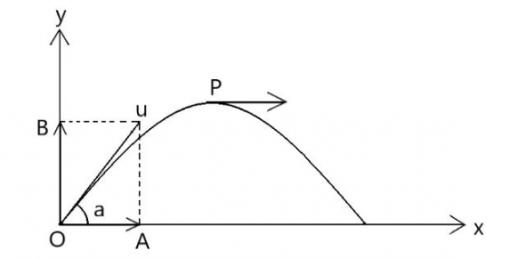# Acceleration, Distance And Time Reasoning Test! Trivia Quiz

6 Questions | Total Attempts: 98SettingsDistance and time are the best way of determining the speed at which an object moves. As agreed the exam below was set up to test your understanding on the topic of acceleration, distance and time. You can do it at home but remember that once the clock stops that is it. Give it a shot!

• 1.
A bullet comes to rest in a block of wood in 1.0 x 10^-2 seconds, with an acceleration of -8.0 x 10^4 metres per second squared.  What was its original speed, in metres per second?
• A.

-800 m/s

• B.

800 m/s

• C.

12.5 m/s

• D.

-12.5 m/s

• 2.
The light turns red, and you come to a screeching halt.  Checking your stopwatch, you see that you stopped in 4.5 seconds.  Your deceleration was 1.23 x 10^-3 miles per second squared.  What was your original speed in miles per hour?
• A.

21.6 miles/hour

• B.

0.006 miles/hour

• C.

0.36 miles/hour

• D.

0.0001 miles/hour

• 3.
A car is going 60 miles per hour and accelerating at 10 miles per hour squared.  How far does it go in 1 hour?
• A.

70 miles

• B.

35 miles

• C.

65 miles

• D.

30 miles

• 4.
A motorcycle is going 60 miles per hour and decelerating at 60 miles per hour squared.  How far does it go in 1 hour?
• A.

90 miles

• B.

60 miles

• C.

30 miles

• D.

120 miles

• 5.
A block of wood is shooting down a track at 10 metres per second and is slowing down because of friction.  If it comes to rest in 20 seconds and 100 metres, what is its deceleration, in metres per second squared?
• A.

-0.5 m/s^2

• B.

0.5 m/s^2

• C.

1.5 m/s^2

• D.

-1.5 m/s^2

• 6.
A car starts from rest and is accelerated at 5.0 metres per second squared.  What is its speed 500 metres later?
• A.

26 m/s

• B.

50 m/s

• C.

5000 m/s

• D.

70.7 m/s

Related TopicsBack to top